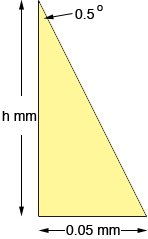I am trying to solve for the height of a right triangle. The base is .05 mm, and the apex is 0.5 degrees. I vaguely recall from high school that this should be enough information to solve for height, but I can't remember what equation(s) to use. Hi Samantha, With the help of a little trigonometry this is enough information to find the height.From the diagram tan(0.5o) = 0.05/h  and hence h = 0.05/tan(0.5)  = 0.05/0.0087  = 5.7 mm Penny